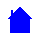## P10C Course Outline

```This page is provided to assist lecturers and students in the other Caribbean islands - so
that they may keep pace with us as we explore the world of Mechanics !

Tutorials and Answer Sheets will be available as we progress

Course Outline:
-----------------------
Lecture 1 -  Motion defined (position, displacement, speed, velocity and acceleration)
Lecture 2 -  Methods of analysis (average, instantaneous, graphical treatment, calculus method)
Lecture 3 -  Vectors and Scalars, vector components and unit vectors, graphical treatment
Lecture 4 -  Mathematical operations on vectors, Dot and Cross Product, importance in Physics
Lecture 5 -  Motion in a plane (2D) and in space (3D)
Lecture 6 -  Projectile motion, Circular motion, reference frames and relative motion
Lecture 7 -  Newtons Laws, Force and Inertia, Mass and Gravity.
Lecture 8 -  Newtons Laws, Friction and Tension
Lecture 9 -  Static and Kinetic frictional forces, drag and terminal speed
Lecture 10 - Circular motion, centripetal force, energy and work
Lecture 11 - Work done by various forces, three dimensional analysis, Power
Lecture 12 - Potential energy and Conservation of Energy
Lecture 13 - Review of all covered so far
Lecture 14 - Class Test
Lecture 15 - Centre of Mass and Linear Momentum
Lecture 16 - Elastic and inelastic collisions in one, two and three dimensions, variable mass
Lecture 17 - Angular position, displacement, velocity and acceleration
Lecture 18 - Kinetic energy, inertia, torque, work and Newtons Laws applied to Rotation
Lecture 19 - Rolling, translation and rotation, forces and energy in rolling
Lecture 20 - Yo-yo, torque and angular momentum in rolling, precession of a gyroscope
Lecture 21 - Gravitation, force and energy in gravitation, escape speed
Lecture 22 - Keplers Laws, orbits of planets and satellites, Einstein's general theory of relativity
Lecture 23 - Velocity, acceleration, force and energy in Simple Harmonic Motion
Lecture 24 - Pendulums, forced oscillation, resonance and damping
Lecture 25 - Review of all material covered
Lecture 26 - Class Test

Recommended text: Fundamentals of Physics (7th Edition) by Halliday, Resnick and Walker
```P10C Homepage